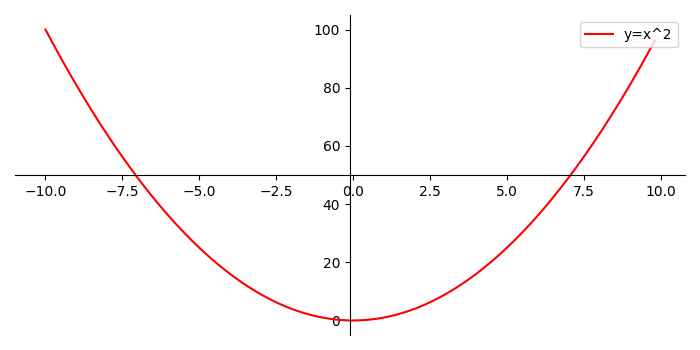# How to draw axis in the middle of a figure in Matplotlib?

To draw axis in the middle of a figure, we can take the following steps −

• Create and sqr data points using numpy.

• Create a new figure, or activate an existing figure, using figure() method.

• Add an axis to the figure as a part of a subplot arrangement.

• Set the postion of left and bottom spines.

• Set the color of the right and top spines.

• Plot x and sqr, using plot() method, with label y=x2 and color=red.

• Place the legend using legend() method. Set the location at upper right corner.

• To display the figure, use show() method.

## Example

import numpy as np
import matplotlib.pyplot as plt
plt.rcParams["figure.figsize"] = [7.00, 3.50]
plt.rcParams["figure.autolayout"] = True
x = np.arange(-10., 10., 0.2)
sqr = np.square(x)
fig = plt.figure()
plt.show()